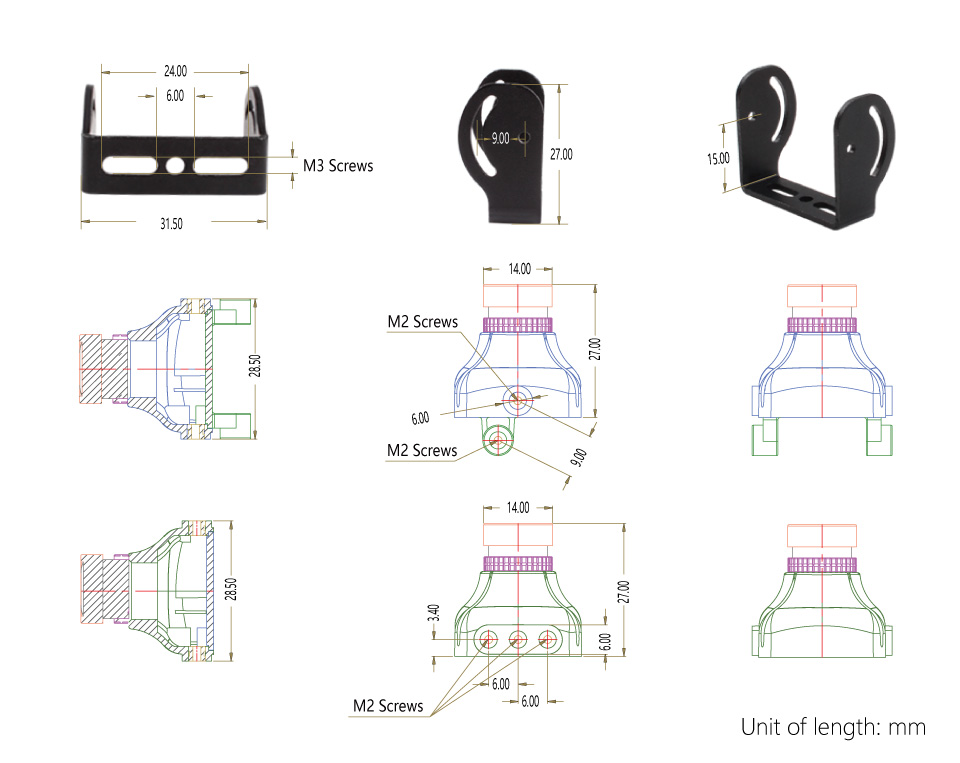# I can’t find the sizes that I need like the placements of the mounting holes of the Swift.

The size of the Swift is as below:1 out of 1 found this helpful

### Have more questions? Submit a request

•gail w selby

This isn't correct for the runcam I got one amazon (2)

•James Stergar

several resources show the main hole to adjustment hole distance as 9mm so the dimensions above do not add up with simple math:

a^2 + b^2 = c^2

7^2 + 4^2 = c^2

49 + 16 = c^2

65 = c^2

sqr(65) = c

c = 8.06mm

So if A and B are 7mm and 4mm then the hole distance is 8.06 so something is off.

•Katie

Thanks to gail and James. The illustration has been updated:)

Edited by Katie